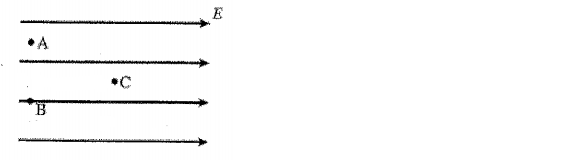# Problem: Suppose a region of space has a uniform electric field, directed toward the right, as shown in the figure. Which statement is true? A) The voltage at all three location is the same. B) The voltage at point A and B are equal, and the voltage at point C is higher than the voltage at point A. C) The voltage at points A and B are equal, and the voltage at point C is lower than the voltage at point A. D) The voltage at point A is the highest, the voltage at point B is the second highest, and the voltage at point C is the lowest. E) None of the above.

###### FREE Expert Solution
82% (393 ratings)
###### Problem Details

Suppose a region of space has a uniform electric field, directed toward the right, as shown in the figure. Which statement is true?

A) The voltage at all three location is the same.

B) The voltage at point A and B are equal, and the voltage at point C is higher than the voltage at point A.

C) The voltage at points A and B are equal, and the voltage at point C is lower than the voltage at point A.

D) The voltage at point A is the highest, the voltage at point B is the second highest, and the voltage at point C is the lowest.

E) None of the above.Frequently Asked Questions

What scientific concept do you need to know in order to solve this problem?

Our tutors have indicated that to solve this problem you will need to apply the Equipotential Surfaces concept. You can view video lessons to learn Equipotential Surfaces. Or if you need more Equipotential Surfaces practice, you can also practice Equipotential Surfaces practice problems.

How long does this problem take to solve?

Our expert Physics tutor, Juan took 1 minute and 37 seconds to solve this problem. You can follow their steps in the video explanation above.

What professor is this problem relevant for?

Based on our data, we think this problem is relevant for Professor Efthimiou's class at UCF.